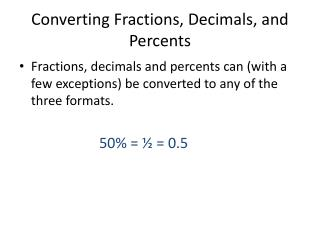DownloadDownload PresentationConverting Fractions, Decimals, and Percents

# Converting Fractions, Decimals, and Percents

Download Presentation## Converting Fractions, Decimals, and Percents

- - - - - - - - - - - - - - - - - - - - - - - - - - - E N D - - - - - - - - - - - - - - - - - - - - - - - - - - -
##### Presentation Transcript

1. Converting Fractions, Decimals, and Percents • Fractions, decimals and percents can (with a few exceptions) be converted to any of the three formats. 50% = ½ = 0.5

2. Converting Fractions, Decimals, and Percents • A fraction to a decimal: Divide the denominator (the bottom part) into the numerator (the top part): 1/4 = 1 ÷ 4 = 0.25

3. Converting Fractions, Decimals, and Percents • A fraction to a percent: First change the fraction to a decimal. Then, move the decimal two places to the right and attach a percent sign. 1/4 = 1 ÷ 4 = .25 = 25%

4. Converting Fractions, Decimals, and Percents • A decimal to a fraction: Starting from the decimal point, count the decimal places. If there is one decimal place, put the number over 10 and reduce (divide the numerator and denominator by the same number). If there are two places, put the number over 100 and reduce. If there are three places, put it over 1000 and reduce, and so on. 0.25 = 25/100 = 1/4

5. Converting Fractions, Decimals, and Percents • A decimal to a percent: Move the decimal point two places to the right. Then, attach a percent sign. 0.25 = 25% • A percent to a decimal: Move the decimal point two places to the left. Then, drop the percent sign. 25% = 0.25

6. Converting Fractions, Decimals, and Percents • A percent to a fraction: Put the number over 100 and reduce. Then, drop the percent sign. 25% = 25/100 = 1/4

7. Converting Fractions, Decimals, and Percents ½ = .50 = 50% ¼ = .25 = 25% ¾ = .75 = 75% ⅕ = .2 = 20% ⅓ = = 33⅓ % ⅔ = = 66⅔ %

8. Converting Fractions, Decimals, and Percents • Note: Fractions that convert to repeating decimals cannot be worked with in decimal form. • 1/3 becomes which is not the same as simply .333! • To accurately convert a repeating decimal to a percent, you need to include the original fraction after the whole percentage. • 1/3 becomes 33⅓%

9. Converting Fractions, Decimals, and Percents • Try the practice on page 141 in the book. • You should now be able to do the GED Practice pages 18 - 21, which includes material from many of the subjects we’ve covered so far. • Submit your answers using the Number Operations answer sheet.